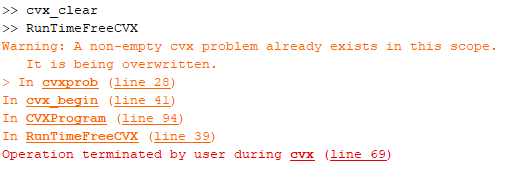# How to remove error message following failure in iteration

Hello, I have a CVX program running that every now and then fails. Upon failure some parameters are tweaked and it is re-ran automatically. Whenever I re-run after a failure I get a large message:

``````Warning: A non-empty cvx problem already exists in this scope.
It is being overwritten.
> In cvxprob (line 28)
In cvx_begin (line 41)
In CVXProgram (line 93)
In RunTimeFreeCVX (line 38)
``````

I would love to know how to close or remove this non-empty CVX problem upon a failure so that my command window isn’t clogged up with these messages.

Thank you!

… … Use `cvx_clear`

I’m so sorry if that was a stupid question, I should have mentioned that I have tried this command and it doesn’t seem to be having the desired effect.

Even if I purposefully stop the process (to cause a failure) and type the command into the command window it appears to clear the cvx problem, but then returns the same message when I start the program.I have no idea what `RunTimeFreeCVX` is or does. That is not a standard part of CVX. if that is your own program, maybe it does its own `overwriting`, thereby triggering the warning message.

If that does not resolve it, please show a complete minimum reproducible example, starting from a fresh MATLAB session, which exhibits the issue. This should be copy and pasted using Preformatted text icon, not an image.

For all intents and purposes all it does is run cvx program for a number of different “final times” so that I can run an algorithm to find the best time (the one that minimizes cost). The code for that is as follows:

``````function [optimal] = RunTimeFreeCVX
cvx_clear
clearstate = false;
Re = 6378e3; % Radius of Earth in m.
g = 9.81;    % Acceleration due to gravity in m/s^2.
xi = [100e3+Re;0*pi/180;0*pi/180;6900;0*pi/180;0*pi/180;0]./[Re;1;1;sqrt(Re*g);1;1;1];
xf = [15e3+Re;15*pi/180;45*pi/180;0;0*pi/180;0*pi/180;0]./[Re;1;1;sqrt(Re*g);1;1;1];

% Empty Preallocation
ts = [];
costs = [];
data = [];
% Time parameters.
t = 1000; % Initial guess.
dt = 500; % Change in time.

% Count initialization
cnt = 0;

% Convergence checker
closeenough = false;

% Max iteration number
maxiter = 100;

% Time-free Program
while numel(ts) < 2 || ~closeenough || strcmp(output.status,'Failed') || strcmp(output.status,'Infeasible') || strcmp(output.status,'Inaccurate/Solved')
if clearstate
clc
end
cnt = cnt+1;
if numel(ts)>2
closeenough = ((ts(end)-ts(end-1))<10);
end
ts = [ts;t];
cvx_clear
try
[output] = CVXProgram(xi,xf,ts(cnt));
costs = [costs;output.cost];
if ~strcmp(output.status,'Failed') && ~strcmp(output.status,'Infeasible') %&& ~strcmp(output.status,'Inaccurate/Solved')
fprintf('Counter: %.0f. Time: %.0f Feasible.\n',cnt,t)
data = [data,output];
else
fprintf('Counter: %.0f. Time: %.0f Infeasible.\n',cnt,t)
end
catch
cvx_clear
close all
costs = [costs;inf];
output.status = 'Failed';
fprintf('Counter: %.0f. Time: %.0f Infeasible.\n',cnt,t)
end
if numel(costs)<2
t = t+dt;
else
lowestcost = min(costs);
cheapert = ts(costs==lowestcost);
if t > cheapert
t = (t-(1/cnt))*dt;
if t <= 0
t = 500;
end
elseif t < cheapert
t = (t+(1/cnt))*dt;
if t >= 10000
t = 10000;
end
else
t = t+dt;
end
end
if cnt>=floor(maxiter/2) && size(data,2) >= 1
if clearstate
clc
end
fprintf('Feasible Time Found. Terminating.')
break
end
if numel(ts)>=maxiter
break
end
end
cost = [];
for i = 1:size(data,2)
cost = [cost;data(i).cost];
end
[~,j] = min(cost);
optimal = data(j);
end
``````

The CVXProgram that is triggered in here is literally just everything from the cvx_begin to the cvx_end, so it is being triggered as it reaches this point (meaning the cvx program is still alive through this process). Sometimes the algorithm or the iterations pick a time that isn’t feasible (for example it may pick a time that is too low for the aircraft to make the journey, or too high to retain sufficient fuel). In these cases the program throws out an error, and the try/catch sorts this out. However, the try/catch keeps the cvx program around and causes the error message the next time I try to use begin_cvx.

1 Like

It sounds like you need to improve the logic of how cvx_clear is integrated with try/catch.

Here is an old post of mine. Detecting of returning error in CVX

1 Like